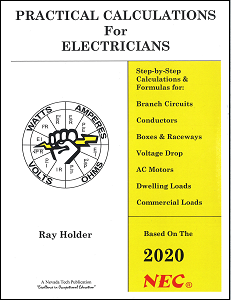#### 2020 PRACTICAL CALCULATIONS FOR ELECTRICIANS## \$47.95 + \$8.50 S/H

2020 PRACTICAL CALCULATIONS FOR ELECTRICIANS

This is THE book every electrician should own.
This comprehensive electrical calculations textbook is based on the 2020 NEC® and contains complete coverage of core concepts of electrical calculations needed by every electrician. This book is arranged with topic-by-topic organization and step-by-step calculation procedures giving the electrician insight and understanding to solving mathematical problems. The text contains 10 main topic units filled with related information, with a Self-Assessment Quiz following each unit, as well as a 90 question final exam. This textbook will familiarize you with formulas and calculations for branch circuits, ac motors, voltage drop, power factor, conductors, boxes and raceways, appliances, dwellings, commercial occupancy, and many more topics. Whether for continuing education or exam preparation, this book is a MUST for every electricians.

294 pages, 8½” x 11″, spiral bound

ISBN: 978-1-935834-21-2